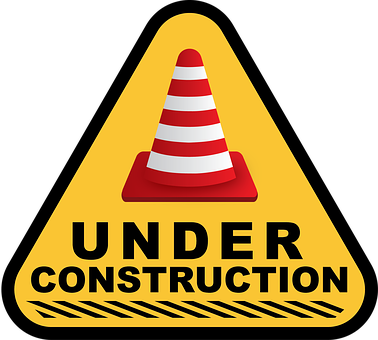### Mathematics involves experimenting and problem-solving!

Welcome
to Mr. Kimble's Math Class. I look forward to teaching you math! If you have any questions or comments, please contact me at akimble@lccsnj.org.

This year students will learn about linear functions, inverse functions, Pythagorean Theorem, exponential functions, logarithmic functions, trigonometric functions, polynomial functions, linear inequalities, absolute functions, quadratic functions, coordinate geometry, symmetry, rotation, symbolic expressions, square roots, cube roots, volume, scientific notation, rational numbers, irrational numbers, radical expressions, domain, range, arithmetic sequence, geometric sequence, etc.

Homework: